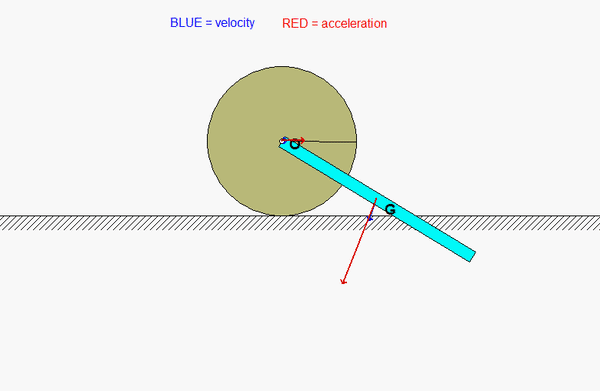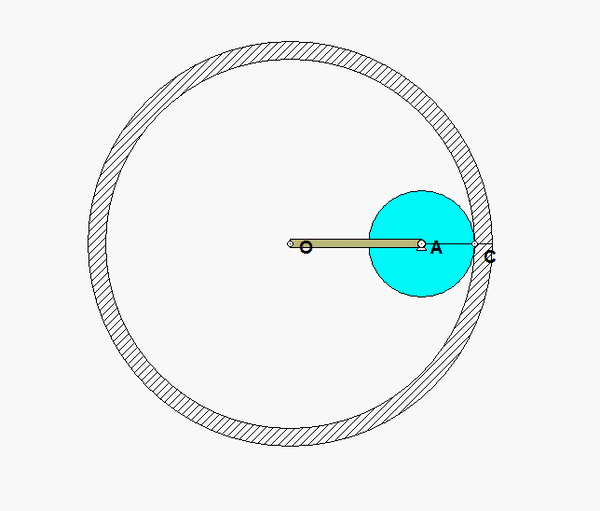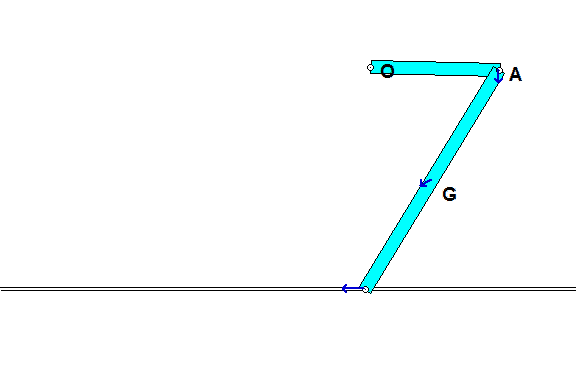# Vibrations. Vibrations. And, more vibrations.

Here in Chapter 6 we are investigating systems that exhibit oscillatory motion. This may seem like a new topic for this course for us. In looking back at lecture book examples and homework problems, we discover that many of these problems have exhibited oscillatory motion. We did not pursue this motion in our analysis, rather we looked at determining accelerations and velocities at particular instants in time or particular positions. You can see many of these examples below.

Our analysis in this chapter will focus on solving differential equations that describe the motion of systems as a function of time. Specifically, we will look at a subset of the systems below that are described by linear equations of motion, systems for which we have the mathematical background to study at your current juncture. Some of the systems below can be described by linear differential equations.